Disclosure: This post may contain affiliate links, meaning when you click the links and make a purchase, we receive a commission.

# How to Separate Words in Excel Using Formula (Ultimate Guide)

Separating words are important in Excel to extract information from your worksheet. There are many methods of doing that. Here, in this article, we will see “How to Separate Words in Excel Using Formula”. We will use many different examples to demonstrate the processes like separate words by the delimiter, separating text from numbers, separate different format names from a text string etc.

## Separate Words by Delimiter using Formula

In this example, we will work with words with the delimiters. Suppose, we have a list of Items along with other information like color and size. These are written in a cell with combinations of the colon. Our goal is to separate these words in different columns to make the information more precise. Let`s see the below table.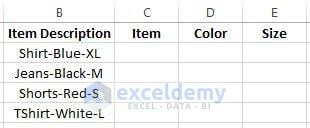In the above table, you can see the description of different items. Here, we create extra 3 columns to extract the exact information in each specific column from the Item Description column.

### Extracting Items from Text Strings

First of all, we are going to extract the item names from the Item Description. The process of doing this is given below,

• Write down the formula, `=LEFT(B2,SEARCH("-",B2,1)-1) `in cell C2.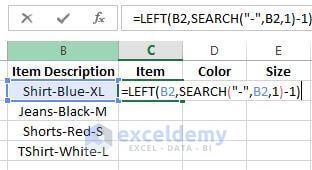• Now press Enter. You will see the item name from cell B2 appears in cell C2.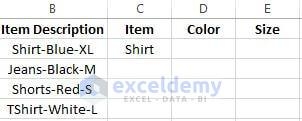• Drag the formulated cell C2 downwards to copy this formula for the rest of the cells of the Item column.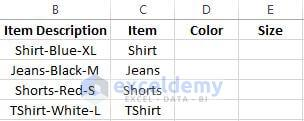### Extracting Colors name from the Text Strings

Now, to extract the color name follow the below steps.

• First, write down the formula,` =MID(B2, SEARCH("-",B2) + 1, SEARCH("-",B2,SEARCH("-",B2)+1) - SEARCH("-",B2) - 1) ` in cell D2.• After pressing Enter you will get to see the below result.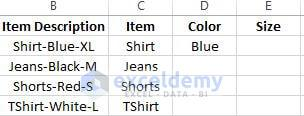Drag the formulated cell D2 downwards to copy this formula for the rest of the Color column.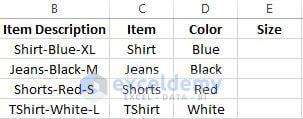### Extracting Size from the Text String

To extract Size we will use a different formula that uses RIGHT, LEN, and SEARCH functions together. The procedure for this is given below.

• Write the formula `=RIGHT(B2,LEN(B2)-SEARCH("-",B2, SEARCH("-",B2) + 1)) `in cell E2.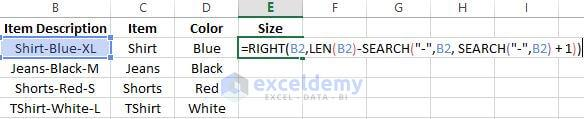• After pressing Enter you will get the below result.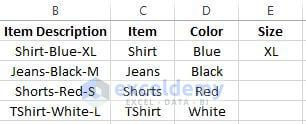• Like earlier, drag the formulated cell downwards to copy this formula for the rest of the cells and you will get the below result.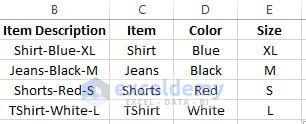## Separating Text from Numbers

In many cases, you will find text with numbers and from that, you may need the separate the texts. There are few formulas for doing that. Let’s look into the below table. From this table, we will extract the cities name and postcodes separately.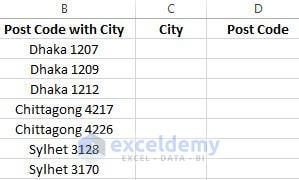### Extracting Words and Numbers by counting Characters

One of the processes of doing that is counting the total digits in a cell and from them extracting the number and word that you want.

• In cell D2, write down the formula, `=RIGHT(B2,SUM(LEN(B2) - LEN(SUBSTITUTE(B2, {"0","1","2","3","4","5","6","7","8","9"},"")))) `and press Enter.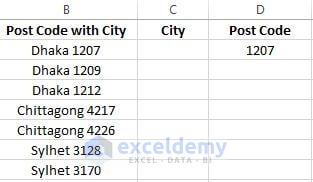• Drag this formulated cell downwards for the rest of the cells of column Post Code.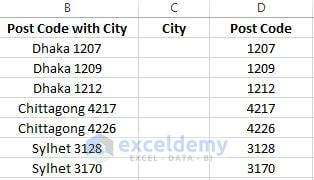• Now to extract Cities name write down the formula, `=LEFT(B2,LEN(B2)-LEN(D2))` in cell C2 and press Enter.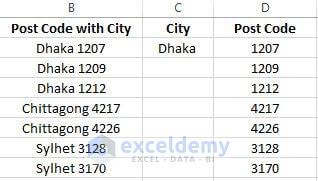• By dragging this cell downwards you will get all the cities name in the City column.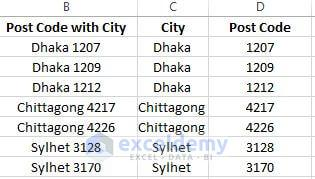### Finding the position of the First digit of a String

This process involves finding the position of the 1st digit of a number in a cell. There will be a separate column to find the position of the 1st digit.

• In cell C2, write down the formula `=MIN(SEARCH({0,1,2,3,4,5,6,7,8,9},B2&"0123456789")) `and press Enter. After this double-click, the Fill Handle option or drag down the formulated from C2 to C8 to copy this formula for the rest of the cell. You will get to see the below result.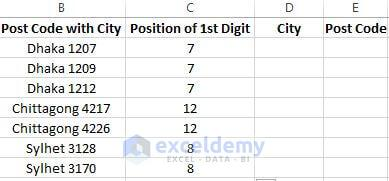• Now to extract cities name write the formula, `=LEFT(B2, C2-1)` in cell D2 and copy this formula for the rest of the cells of Column City. You will get the below result after that.• For extracting the numbers use the formula `=RIGHT(B2, LEN(B2)-C2+1) `in cell E2 and drag it down for the rest of the cells of column Postcode.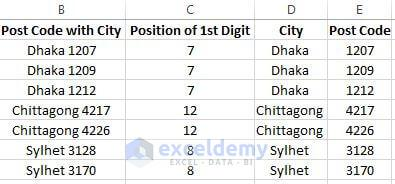## Separate Words in Excel Using Formula (Working With Different Names)

In this example, we will be working different format names. From this names, we will be extracting first names, middle names, last names, suffixes of names. Let`s look into the below picture so that you will get an idea of what we are going to do.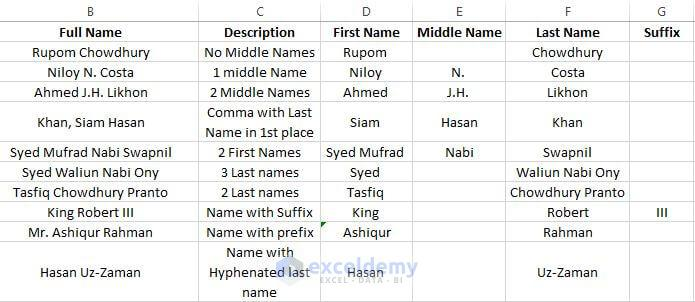### Separating Names from a Cell Which Has “No Middle Names”

Here we are going to extract the 1st and last name from a cell which has no middle names. Look into the below table to get a clear idea about the formulas and the results.

 Full Name Rupom Chowdhury Description No Middle Names Formula For the First Name `=LEFT(B2, SEARCH(" ",B2,1))` First Name Result Rupom Formula For the Last Name `=RIGHT(B2,LEN(B2)-SEARCH(" ",B2,1))` Last Name Result Chowdhury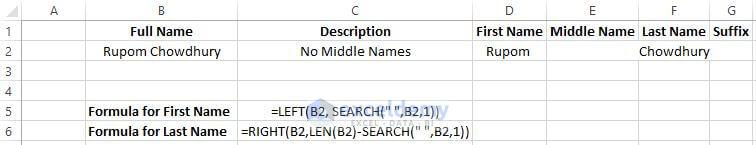### Extracting Names with “1 Middle Name”

In this example, you are going to see the extraction of 3 names from a cell. Follow the procedure given in the below table for getting the desired result.

 Full Name Niloy N. Costa Description 1 Middle Name Formula For the First Name `=LEFT(B2, SEARCH(" ",B2,1))` First Name Result Niloy Formula For the Middle name `=MID(B2,SEARCH(" ",B2,1)+1,SEARCH(" ",B2,SEARCH(" ",B2,1)+1)-SEARCH(" ",B2,1))` Middle Name Result N. Formula for the Last Name `=RIGHT(B2,LEN(B2)-SEARCH(" ",B2,SEARCH(" ",B2,1)+1))` Last Name Result Costa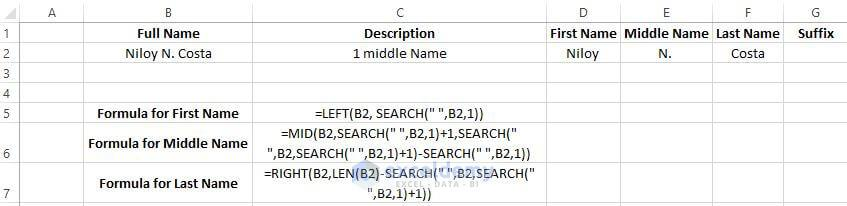### Extracting Names with “2 Middle Names”

This example shows the extraction of names having 2 middle names. The first and last names will also be extracted along with the two middle names.

 Full Name Ahmed J.H. Likhon Description 2 Middle Names Formula For the First Name `=LEFT(B2, SEARCH(" ",B2,1))` First Name Result Ahmed Formula For the Middle name `=MID(B2,SEARCH(" ",B2,1)+1,SEARCH(" ",B2,SEARCH(" ",B2,SEARCH("",B2,1)+1)+1)-SEARCH(" ",B2,1))` Middle Name Result J.H. Formula for the Last Name `=RIGHT(B2,LEN(B2)-SEARCH(" ",B2,SEARCH("",B2,SEARCH(" ",B2,1)+1)+1))` Last Name Result Likhon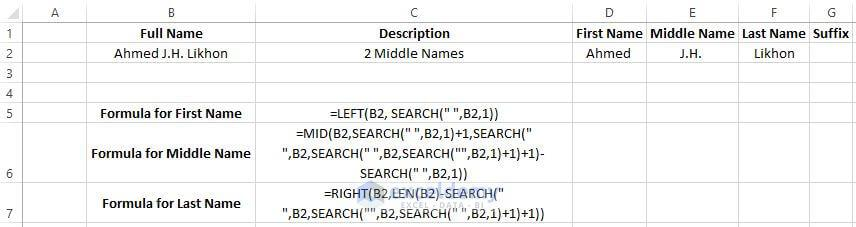### Separating Names from USA Name Format (Comma with the Last Name in 1st place)

If any name is written in the USA name format you can extract the first, last and middle names by following the formulas stated in the below table.

 Full Name Khan, Siam Hasan Description Comma with the Last Name in 1st Place Formula For the First Name `=MID(B2,SEARCH(" ",B2,1)+1,SEARCH(" ",B2,SEARCH(" ",B2,1)+1)-SEARCH(" ",B2,1))` First Name Result Siam Formula For the Middle name `=RIGHT(B2,LEN(B2)-SEARCH(" ",B2,SEARCH(" ",B2,1)+1))` Middle Name Result Hasan Formula for the Last Name `=LEFT(B2, SEARCH(" ",B2,1)-2)` Last Name Result Khan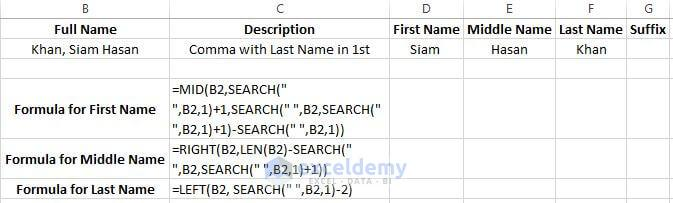## Separating Names from Text string with “2 First Names”

To extract names with the first name having 2 names follow the formula stated in the below table.

 Full Name Syed Mufrad Nabi Swapnil Description 2 First Names Formula For First Name `=LEFT(B2, SEARCH(" ",B2,SEARCH(" ",B2,1)+1))` First Name Result Syed Mufrad Formula For the Middle name `=MID(B2,SEARCH(" ",B2,SEARCH(" ",B2,1)+1)+1,SEARCH(" ",B2,SEARCH(" ",B2,SEARCH(" ",B2,1)+1)+1)-(SEARCH(" ",B2,SEARCH(" ",B2,1)+1)+1))` Middle Name Result Nabi Formula for the Last Name `=RIGHT(B2,LEN(B2)-SEARCH(" ",B2,SEARCH(" ",B2,SEARCH(" ",B2,1)+1)+1))` Last Name Result Swapnil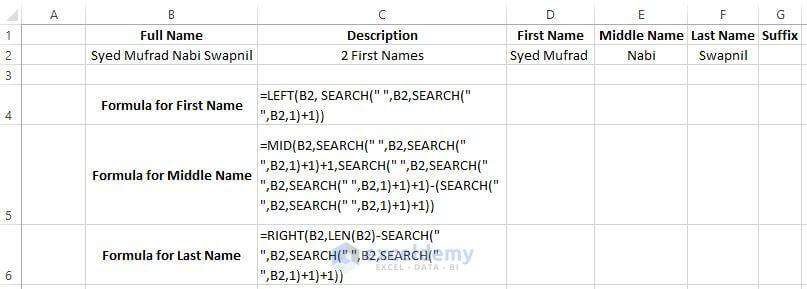### Separating Names from Text string with “3 Last Names”

Use the formula given in the below table to extract first and last name which has 3 names as the last name.

 Full Name Syed Waliun Nabi Ony Description 3 Last Names Formula For the First Name `=LEFT(B2, SEARCH(" ",B2,1))` First Name Result Syed Formula For the Last Name `=RIGHT(B2,LEN(B2)-SEARCH(" ",B2,1))` Last Name Result Waliun Nabi Ony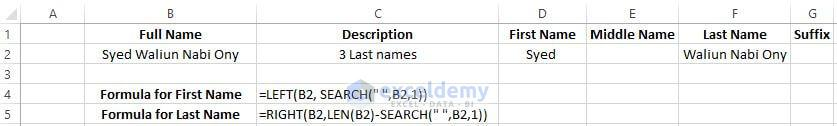### Separating Names from Text string with “2 Last Names”

Use the formula given in the below table to extract first and last name which has 2 names as last name.

 Full Name Tasfiq Chowdhury Pranto Description 2 Last Names Formula For the First Name `=LEFT(B2, SEARCH(" ",B2,1))` First Name Result Tasfiq Formula For the Last Name `=RIGHT(B2,LEN(B2)-SEARCH(" ",B2,1))` Last Name Result Chowdhury Pranto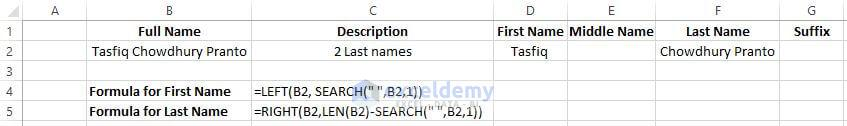### Separating names with “Suffix”

Names which contain suffix can be extracted by using the formulas stated in the below table.

 Full Name King Robert III Description Suffix Formula For the First Name `=LEFT(B2, SEARCH(" ",B2,1))` First Name Result King Formula For the Last name `=MID(B2,SEARCH(" ",B2,1)+1,SEARCH(" ",B2,SEARCH(" ",B2,1)+1)-(SEARCH(" ",B2,1)+1))` Last Name Result Robert Formula for Suffix `=RIGHT(B2,LEN(B2)-SEARCH(" ",B2,SEARCH(" ",B2,1)+1))` Suffix Result III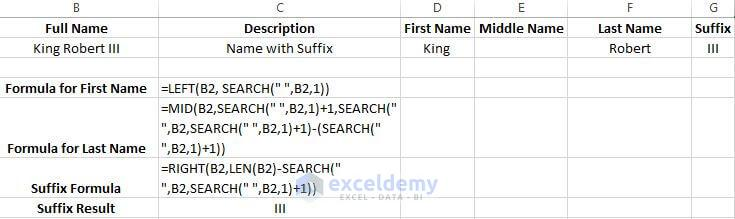### Separating Names without “Prefix”

To ignore prefix in a name while extracting the First and last name you can use the below formulas.

 Full Name Mr. Ashiqur Rahman Description Names with Prefix Formula For the First Name `=MID(B2,SEARCH(" ",B2,1)+1,SEARCH(" ",B2,SEARCH(" ",B2,1)+1)-(SEARCH(" ",B2,1)+1))` First Name Result Ashiqur Formula For the Last Name `=RIGHT(B2,LEN(B2)-SEARCH(" ",B2,SEARCH(" ",B2,1)+1))` Last Name Result Rahman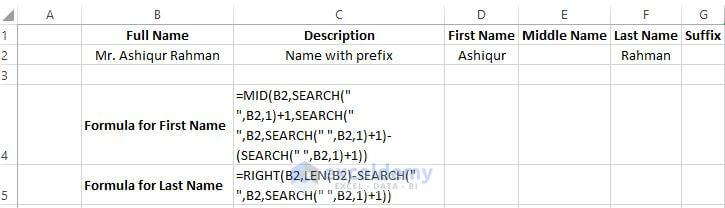### Separating names with “Hyphenated last Names”

Some people names contain hyphens. It is difficult sometimes to extract the hyphenated names. You can easily extract the hyphenated last name by using the formula in the table.

 Full Name Hasan Uz-Zaman Description Name with Hyphenated last name Formula For the First Name `=LEFT(B2, SEARCH(" ",B2,1))` First Name Result Hasan Formula For the Last Name `=RIGHT(B2,LEN(B2)-SEARCH(" ",B2,1))` Last Name Result Uz-Zaman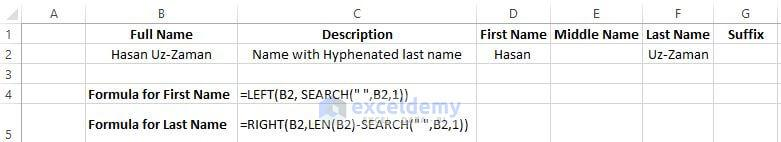## Conclusions

As we can see there are a lot of methods to separate words in Excel using formula. For extracting words in different column Excel has a feature named Text to Column. When you want to extract names from a whole column to different columns you can easily use the Text to Column feature but while working on some specific cells you need to use the formula to extract words.

## Our Recommended Excel Courses

2. Excel Formulas and Functions: Make Basic & Advanced Formulas (Free for my readers)
3. Microsoft Excel – Data Analysis with Excel Pivot Tables (Affiliate link)

## Related Articles

Hello!

Welcome to my Profile. Here I will be posting articles related to Microsoft Excel. I have completed my BSc in Electrical and Electronic Engineering from American International University-Bangladesh. I am a diligent, goal-oriented engineer with an immense thirst for knowledge and attitude to grow continuously. Continuous improvement and life-long learning is my motto.

1. ReplyMadhab Basak Apr 21, 2019 at 1:15 PM

I like very much your way of teaching excel, which is very useful tips often.
.

• ReplyApr 22, 2019 at 9:43 PM

Nice to hear that it helped you. Thanks and regards.

2. ReplyStevoisiak Jul 25, 2019 at 7:49 AM

What if we have both a prefix and a middle name?